# Algebra 1 : How to find percentage from a fraction

## Example Questions

← Previous 1 3 4 5

### Example Question #1 : How To Find Percentage From A Fraction

If Jessica got 2 out of 20 questions wrong on her most recent math quiz, what percent did she get correct?

90%

80%

85%

20%

10%

90%

Explanation:

Since she got 2 out of 20 incorrect, we can first figure out the percent incorrect. We can either find the decimal for 2/20, or make 2/20 a fraction with 100 in the denominator. In this case, it is simpler to do the latter. We need to multiply 20 by 5 to get 100, thus we multiply 2 by 5 to get 10. Then, we have that 2/20 is equivalent to 10/100. She lost 10 percent on her math quiz, leaving her with a score of 90%.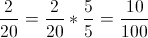10/100 = 10% wrong

(100%) – (10% wrong) = 90% right

### Example Question #2 : How To Find Percentage From A Fraction

Which of the following represents the fraction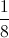as a percentage?

12.5%

10.5%

80%

8%

18%

12.5%

Explanation:

We need to set up a proportion to convert our fraction to x/100.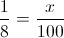Cross multiply.

(1)(100) = (8)(x)

100 = 8x

Divide both sides by 8.

(100)/8 = (8x)/8

100/8 = x

Simplify.

100/8 = 25/2 = 12.5

### Example Question #1 : How To Find Percentage From A Fraction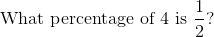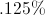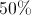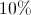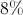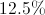Explanation: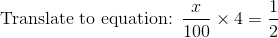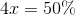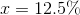### Example Question #2 : How To Find Percentage From A Fraction

A die was rolled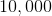times and landed on the number ""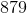times. What percent of the time was the result a number other than?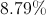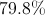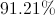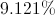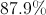Explanation:

Here, the most important step is to understand what the question is asking.

A "" was rolledof a total oftimes, but the question is asking about numbers other than.

A number other thanwas rolled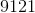times of the total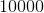.

Now, the final step is to convert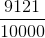into a percent. Sinceis a multiple of, it is a quick conversion.is equal to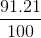and the answer is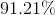.

### Example Question #5 : How To Find Percentage From A Fraction

Judy says she hasof her 8-page paper completed.  What percentage of the paper has she written?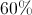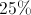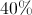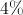Explanation:

Use long division to divide out the fraction to determine the percentage: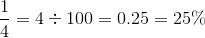### Example Question #1 : How To Find Percentage From A Fraction

A very large bag is filled with marbles.of the bag is filled with red marbles. Anotherof the bag is filled with blue marbles. The rest of the bag is filled with yellow marbles. What percentage of the bag is filled with yellow marbles?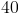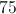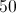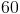Explanation:

First, you must find what fraction of the bag is filled with yellow marbles. We know thatof the bag filled with red marbles andof the bag is filled with blue marbles. So, the fraction of the bag filled with yellow marbles must equal to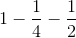orNext, you convertto a percentage, which is 25%.

### Example Question #1 : How To Find Percentage From A Fraction

Jamie ateof a pizza for lunch andof the same pizza later for dinner. What percentage of the pizza is left uneaten?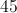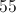Explanation:

Jamie ateof a pizza for lunch andof the same pizza for dinner. To convert a fraction to a percentage, you simply convert the fraction so that the denominator is 100. So, Jamie ate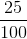of the pizza for lunch and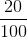of the pizza for dinner. Since the denominator of these fractions is 100, the numerator is its percentage equivalent. Thus, Jamie ate 25% of the pizza for lunch and 20% for dinner for a total of 45% of the pizza. 55% of the pizza remains uneaten.

### Example Question #8 : How To Find Percentage From A Fraction

Billy empties his pockets and finds some change. He finds 2 quarters, 4 dimes, 5 nickels and 9 pennies. What percentage of the coins are nickels?

45%

10%

20%

35%

25%

25%

Explanation:

First, we can find out the proportion of coins that are nickels. So, we know there are 5 nickels and we can count there are 20 coins total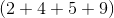So there are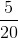coins that are nickels. But the question asks for the percentage. To find the percentage, we can just find our answer as a decimal value rather than a fraction. Than multiply by 100%. So,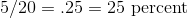### Example Question #9 : How To Find Percentage From A Fraction

A student took a test and got 13 out of 20 questions correct. What percentage of questions did this student get correct?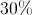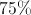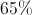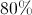Explanation:

We can mathematically write '13 out of 20' as a fraction.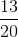We can convert this to a percent by setting up a proportion.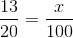Cross multiply and solve for.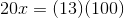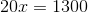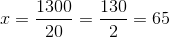So, the student got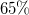of the questions correct.

### Example Question #10 : How To Find Percentage From A Fraction

Write the following fraction as a percent25.1%

51.2%

125%

12.5%

12%

12.5%

Explanation:

To find the percent, first, set up the fraction as a long division problem where the 1 is placed inside the dividend and 8 is placed in the divisor: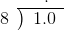Here, the decimal and the zero are included in the division.

Ignoring the decimal in the dividend and treating 1.0 equal to 10, we know that 8 divides into 10 one time.  Therefore, place that one on the top as shown below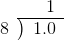Multiply that one by the divisor (8) to get eight (1 x 8 = 8) and then place that eight below the dividend as shown below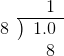Subtract 8 from 10, which will give you 2. That 2 is placed at the bottom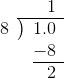To perform the division further, put in an additional zero in the dividend and another zero right beside the 2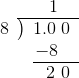This time, we have a 20 at the bottom. 8 can go into 20 two times so we place the two at the top of the (right next to 1) as shown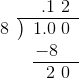Multiply that two by 8 to get sixteen and place that sixteen at the bottom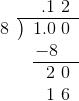Perform the usual subtraction to get 4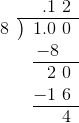Place another zero inside the dividend and another zero right beside 4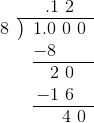This time 8 goes into 40 just perfectly (no remainder)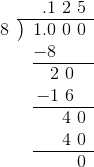The decimal of the fractionis 0.125.  To convert this to a percentage, move the decimal point two places to the right and then put in the '%' symbol so that 0.125 is written as 12.5%

← Previous 1 3 4 5